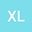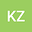Experimental study on the variation mechanism of velocity correction coefficient of overland flow
••• Xiangru Lv,
• kuandi zhang
Xiangru Lv
Northwest A&F University
Author Profilekuandi zhang
Northwest A&F University
Author Profile## Abstract

The velocity correction coefficient is the key to calculate the mean velocity of overland flow, which is a parameter of slope soil erosion process model. Therefore, this paper systematically studied the variation mechanism of the correction coefficient under 5 kinds of slope, 5 kinds of flow rate, 5 kinds of roughness, 9 kinds of gravel coverage and 3 kinds of rainfall intensity conditions based on fixed-bed resistance and simulated rainfall test. The results showed that the relationship between the coefficient and the slope was the inverse function, and its influence on the correction coefficient was weak when the slope was steeper than 10°; both Reynolds number and rainfall intensity could promote the increase of correction coefficient; the relationship between correction coefficient and the submergence degree was in the form of logarithm function, which was alos the same as Reynolds number, but the response of the correction coefficient to both was just opposite. The research results provide the theoretical basis for the accurate selection of velocity correction coefficient, which has important theoretical and practical significance for the investigation of soil erosion power and sediment carrying mechanism of overland flow related to the mean velocity.